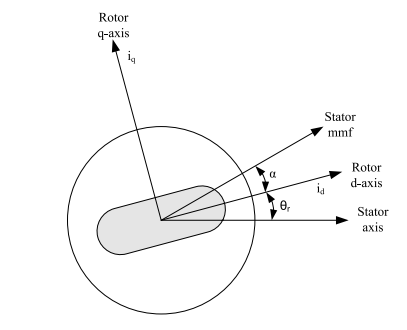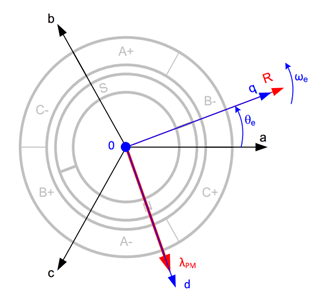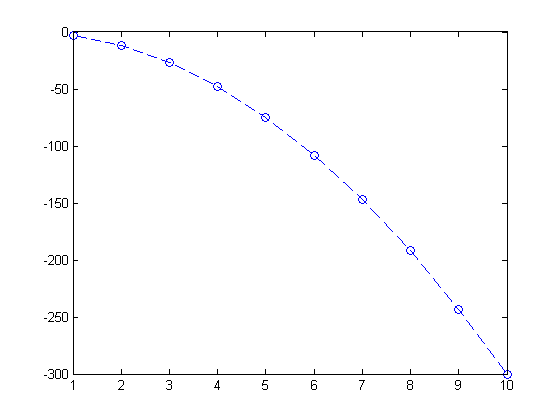# DYNAMIC MODELING OF AC MACHINES

The development and simulation of the dynamic model of a permanent magnet synchronous machine in rotor reference is discussed in this paper. The system model is developed without prebuilt blocks using PSIM software. The results are analysed by varying initial phase of the three-phase voltage source from 0deg to 360 deg. Also the effects on output parameters when fluxlink between stator and rotor is reduces are also discussed.

## Introduction

Ever since the price of high field strength materials particularly neodymium-iron-boron (NdFeB) magnets have decreased in international market, Permanent magnet synchronous motors (PMSM) with the sinusoidal back EMF gained importance because of their high speed, high power density and high efficiency. These properties of PMSM are suitable in special high performance applications, such as; robotics, aerospace, wind turbines, electric ship propulsion systems and hybrid vehicles.

>

## Permanent Magnet Synchronous Motor Model

Using Park's d-q model, the PMSM machines are usually modelled in rotor reference frame. While modelling the equations the following assumptions are made.

• Saturation is neglected.
• The induced EMF is sinusoidal.
• Eddy currents and hysteresis losses are negligible.
• There are no field current dynamics.
• There is no cage on the rotor.

### Figure1: Motor Axis### The stator d, q equations (using Mathjax)

With the above assumptions, the stator d, q equations of the PMSM in the rotor reference frame are-

$$V_q = R_s i_q + \rho\alpha_q + \omega_s\alpha_d$$ $$V_d = R_s i_d + \rho\alpha_d - \omega_s\alpha_q$$

where,

$$\alpha_q = L_q i_q$$

and

$$\alpha_d = L_d i_d + \alpha_{PM}$$

where.

$$V_q \ and \ V_d \ are\ q-axis\ and\ d-axis\ voltages. \nonumber$$, $$i_q \ and \ i_d \ are\ q-axis\ and\ d-axis\ currents. \nonumber$$, $$\alpha_d \ and \ \alpha_q \ are\ the\ d, q\ axis\ stator\ flux\ linkages.\nonumber$$ $$R_s \ stator\ resistance\nonumber$$ $$\alpha_{PM}\ flux\ linkage\ due\ to\ the\ rotor\ magnets\ linking\ the\ stator\nonumber$$. $$\rho \ is\ a\ time\ derivative\nonumber$$

### Figure2: Rotor reference phasor diagram# Sample Matlab publish to html

Initialize the parameters

x=1:1:10;
n=numel(x);
y=zeros(1,n);

%Compute using for-loop
for k=1:n
y(k)=-3*x(k)^2;
end
%Plotting the figure
plot(x,y,'--o')
%Results
x
y

x =

1     2     3     4     5     6     7     8     9    10

y =

-3   -12   -27   -48   -75  -108  -147  -192  -243  -300